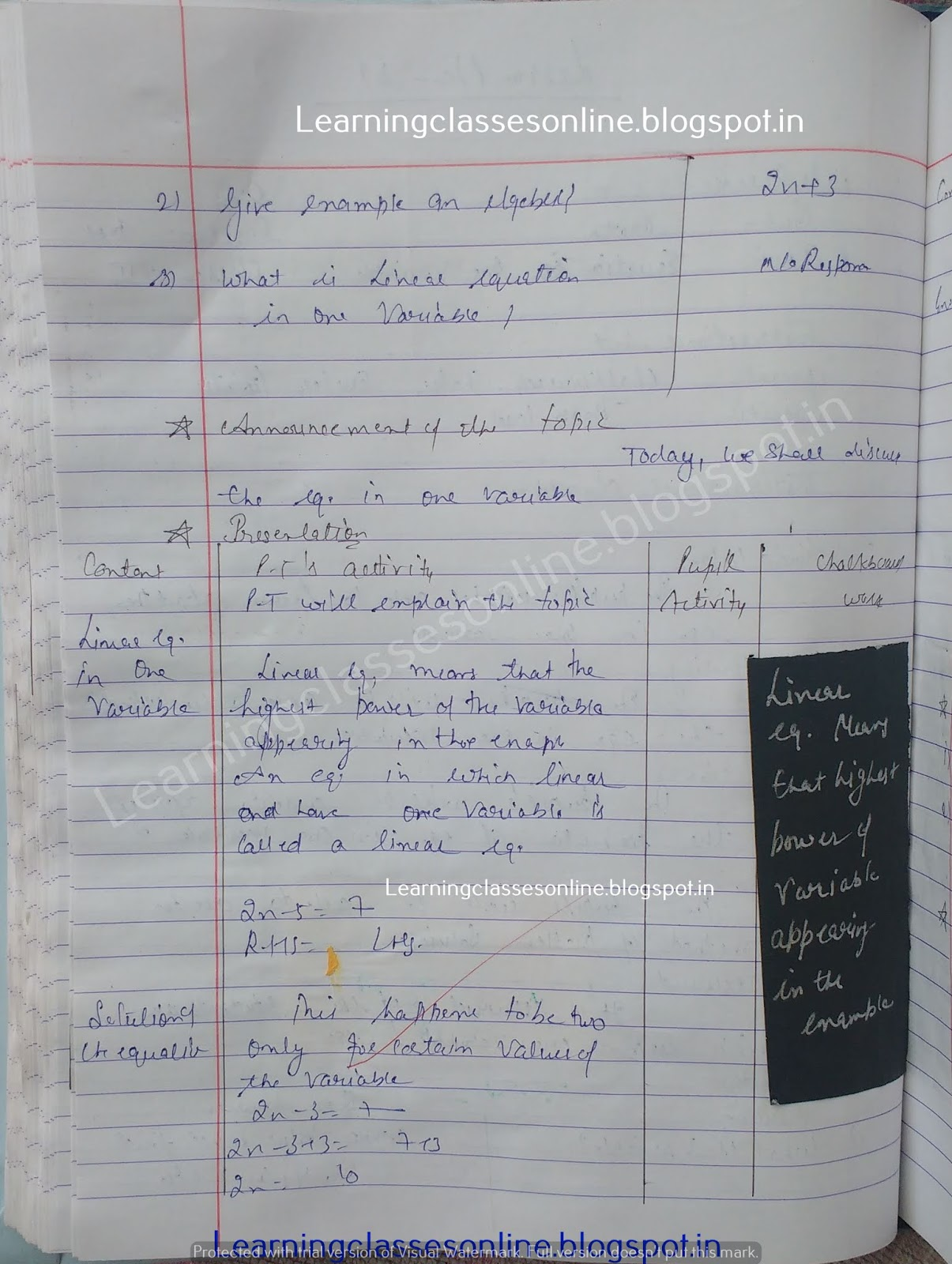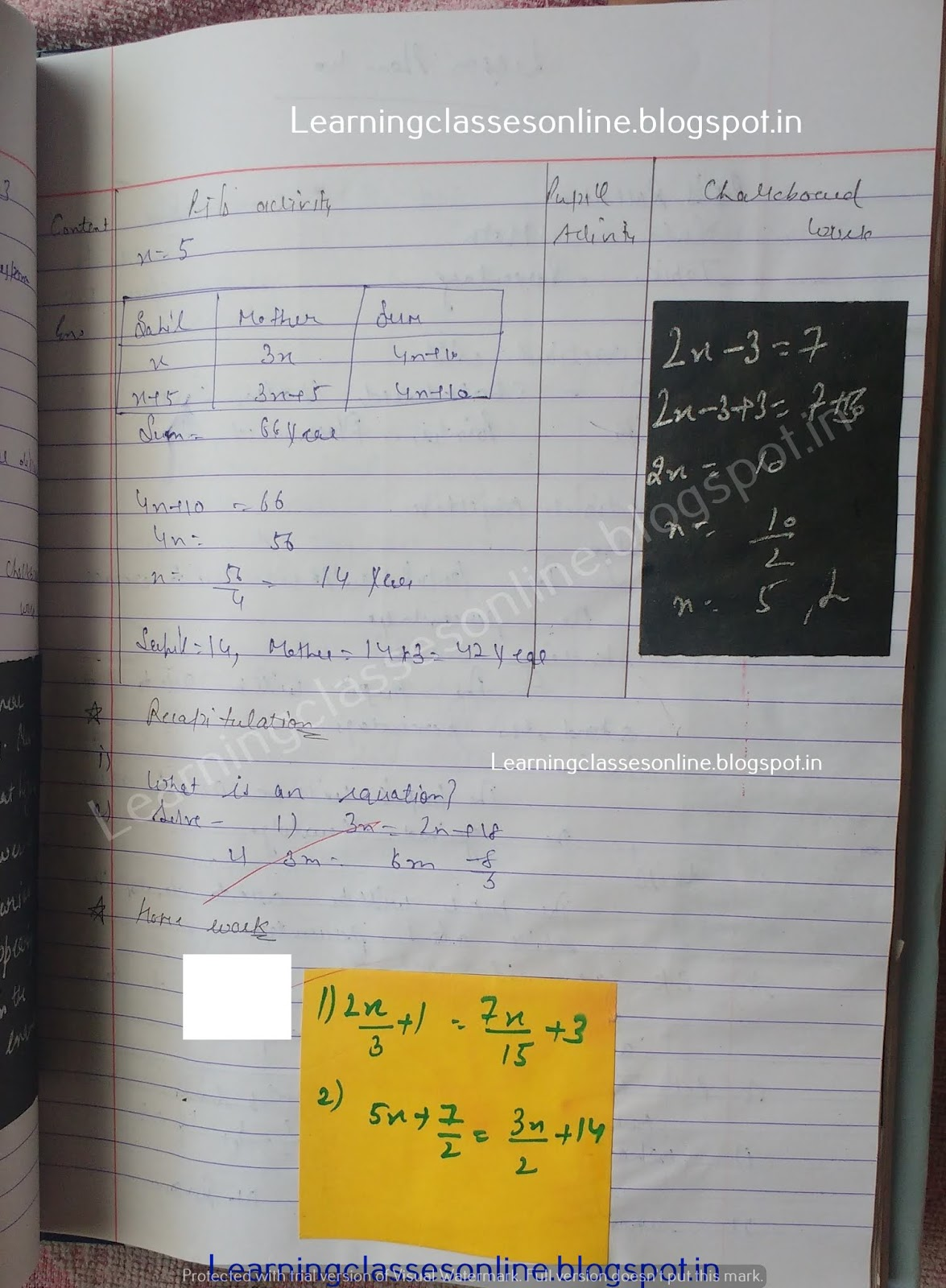Math Lesson Plan on Equation in one Variable

# Math Lesson Plan on Equation in one Variable

Math Lesson Plan on Equation in one Variable for class 6, 7 , 8 and 9

Note: The Mathematics Lesson Plan given below is just an example. You can change the Name, Class, Course, Date, Duration, etc. according to your needs.Further Reference:
www.learningclassesonline.com Mathematics Lesson Plan for B.Ed